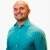# SAT Prep Learn how to write the expression for a word problemThis lesson will look at an example problem involving word problems. This lesson will give an explanation of how to write the expression for a word problem with a step by step look to better explain... This lesson will look at an example problem involving word problems. This lesson will give an explanation of how to write the expression for a word problem with a step by step look to better explain the concept.
More... Collapse
6 Views